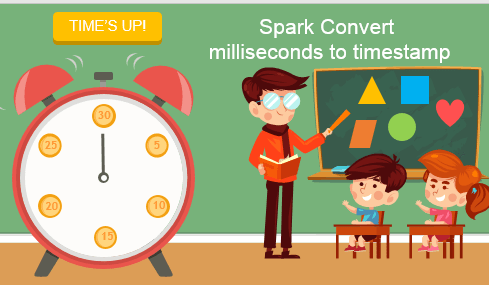# Spark Convert Unix Epoch Seconds to Timestamp

In this article, you will learn how to convert Unix epoch seconds to timestamp and timestamp to Unix epoch seconds on the Spark DataFrame column using SQL Functions with Scala examples.

NOTE: One thing you need to know is Unix epoch time in seconds does not hold milliseconds. hence, it’s not possible to extract milliseconds from Unix time.

Related: Refer to Spark SQL Date and Timestamp Functions for all Date & Time functions.

First, let’ create a DataFrame with current_timestamp() which gives current time and unix_timestamp() which also gives a current time but in Unix epoch seconds.

``````
import org.apache.spark.sql.functions._
val df = Seq(1).toDF("seq").select(
current_timestamp().as("current_time"),
unix_timestamp().as("epoch_time_seconds")
)

df.printSchema()
df.show(false)
``````

Yields below output.

``````
// Output:
root
|-- current_time: timestamp (nullable = false)
|-- epoch_time_seconds: long (nullable = true)

+-----------------------+------------------+
|current_time           |epoch_time_seconds|
+-----------------------+------------------+
|2019-12-23 15:00:36.896|1577142036        |
+-----------------------+------------------+
``````

## 1. Convert Unix Epoch Seconds to Timestamp

Once we have a Spark DataFrame with current timestamp and Unix epoch seconds, let’s convert the “epoch_time_seconds” column to the timestamp by casting seconds to TimestampType.

``````
import org.apache.spark.sql.functions._
// Convert epoch_seconds to timestamp
df.select(
col("epoch_time_seconds").cast(TimestampType).as("current_time"),
col("epoch_time_seconds").cast("timestamp").as("current_time2")
).show(false)
``````

Yields below output.

``````
// Output:
+-------------------+-------------------+
|current_time       |current_time2      |
+-------------------+-------------------+
|2019-12-23 15:00:37|2019-12-23 15:00:37|
+-------------------+-------------------+
``````

## 2. Convert Timestamp to Unix Epoch Time

In this section let’s convert timestamp columns to Unix epoch time column using two ways, first using unix_timestamp() function where it takes a timestamp column as an argument and returns Unix epoch time and second by casting timestamp column to LongType.

``````
import org.apache.spark.sql.functions._
// Convert timestamp to epoch seconds
df.select(
unix_timestamp(col("current_time")).as("unix_epoch_time"),
col("current_time").cast(LongType).as("unix_epoch_time2")
).show(false)
``````

Yields below output.

``````
// Output:
+-----------------+----------------+
|unix_epoch_time  |unix_epoch_time2|
+-----------------+----------------+
|1577142037       |1577142037      |
+-----------------+----------------+
``````

## 4. Source code for reference

``````
package com.sparkbyexamples.spark.dataframe.functions.datetime

import org.apache.spark.sql.SparkSession
import org.apache.spark.sql.functions._
import org.apache.spark.sql.types.{LongType, TimestampType}

object TimeInMilli extends App{

val spark:SparkSession = SparkSession.builder()
.master("local")
.appName("SparkByExamples.com")
.getOrCreate()
spark.sparkContext.setLogLevel("ERROR")

import spark.sqlContext.implicits._

val df = Seq(1).toDF("seq").select(
current_timestamp().as("current_time"),
unix_timestamp().as("epoch_time_seconds")
)

df.printSchema()
df.show(false)

// Convert epoch_time to timestamp
df.select(
col("epoch_time_seconds").cast(TimestampType).as("current_time"),
col("epoch_time_seconds").cast("timestamp").as("current_time2")
).show(false)

// Convert timestamp to Unix epoch time
df.select(
unix_timestamp(col("current_time")).as("unix_epoch_time"),
col("current_time").cast(LongType).as("unix_epoch_time2")
).show(false)

}
``````

The complete code is available at GitHub project for reference

## Conclusion

In this article, you have learned how to convert timestamp to Unix epoch time using `unix_timestamp()` function and Unix Epoch time to timestamp using a cast on the DataFrame column with Scala example.

### Naveen (NNK)

Naveen (NNK) is a Data Engineer with 20+ years of experience in transforming data into actionable insights. Over the years, He has honed his expertise in designing, implementing, and maintaining data pipelines with frameworks like Apache Spark, PySpark, Pandas, R, Hive and Machine Learning. Naveen journey in the field of data engineering has been a continuous learning, innovation, and a strong commitment to data integrity. In this blog, he shares his experiences with the data as he come across. Follow Naveen @ @ LinkedIn

### This Post Has 5 Comments

1.Philip Tellis

Thanks for the useful article, it’s helpful to me, but note that the timestamps you have, ie, 1577142037 and 1577142037 are in seconds, not milliseconds.

1.NNK

Glad it helped you and appreciate for feedback. I’ve corrected it now.

2.Haymang Ahuja

Can someone help on how to extract milliseconds from timestamp, if unix_timestamp does not hold milliseconds.
What is the way around?

3.Haymang Ahuja

In that case where unix_timestamp does not hold milliseconds, then how to extract milliseconds?
For example – How to extract milliseconds from now()
select now(),
from_unixtime(unix_timestamp(now(), ‘yyyy-MM-dd HH:mm:ss.ms’),’hh:MM:ss:ms’) as milli

I have an attribute which holds timestamp in milliseconds and need to rank it based on that. Kindly help

4.Haymang Ahuja

If unix_timestamp does not hold milliseconds, then how to extract the same?
Let’s say I need to extract milliseconds from returned by now()

For example – select now(),
from_unixtime(unix_timestamp(now(), ‘yyyy-MM-dd HH:mm:ss.ms’),’hh:MM:ss:ms’) as milli

I have an attribute holding timestamp till milliseconds, and I need to rank it based on that. Kindly help